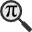## General

Display information for equation id:math.17023.4 on revision:17023

* Page found: Ballistic limit (eq math.17023.4)

(force rerendering)

Cannot find the equation data in the database. Fetching from revision text.

Occurrences on the following pages:

Hash: 14c1fbbbbfd081aaee80274d6468fb9a

TeX (original user input):

\sigma_e\,


TeX (checked):

\sigma _{e}\,


### LaTeXML (experimental; uses MathML) rendering

MathML (950 B / 374 B) :${\displaystyle\sigma_{e}\,}$
<math xmlns="http://www.w3.org/1998/Math/MathML" id="p1.1.m1.1" class="ltx_Math" alttext="{\displaystyle\sigma_{e}\,}" display="inline">
<semantics id="p1.1.m1.1a">
<msub id="p1.1.m1.1.4a" xref="p1.1.m1.1.4.cmml">
<mi id="p1.1.m1.1.1" xref="p1.1.m1.1.1.cmml">σ</mi>
<mi id="p1.1.m1.1.2.1" xref="p1.1.m1.1.2.1.cmml">e</mi>
</msub>
<annotation-xml encoding="MathML-Content" id="p1.1.m1.1b">
<apply id="p1.1.m1.1.4.cmml" xref="p1.1.m1.1.4">
<csymbol cd="ambiguous" id="p1.1.m1.1.4.1.cmml" xref="p1.1.m1.1.4">subscript</csymbol>
<ci id="p1.1.m1.1.1.cmml" xref="p1.1.m1.1.1">𝜎</ci>
<ci id="p1.1.m1.1.2.1.cmml" xref="p1.1.m1.1.2.1">𝑒</ci>
</apply>
</annotation-xml>
<annotation encoding="application/x-tex" id="p1.1.m1.1c">{\displaystyle\sigma_{e}\,}</annotation>
</semantics>
[/itex]


SVG (1.703 KB / 877 B) :

### MathML with SVG or PNG fallback (recommended for modern browsers and accessibility tools) rendering

SVG image empty. Force Re-Rendering

SVG (0 B / 8 B) :

PNG (0 B / 8 B) :

$\sigma _{e}\,$## Translations to Computer Algebra Systems

### Translation to Maple

In Maple: sigma[e]

e: the mathematical constant e == Napier's constant == 2.71828182845... was translated to: e

exp(1): You use a typical letter for a constant [the mathematical constant e == Napier's constant == 2.71828182845...].

We keep it like it is! But you should know that Maple uses exp(1) for this constant.

If you want to translate it as a constant, use the corresponding DLMF macro \expe

### Translation to Mathematica

In Mathematica: Subscript[\[Sigma], e]

E: You use a typical letter for a constant [the mathematical constant e == Napier's constant == 2.71828182845...].

We keep it like it is! But you should know that Mathematica uses E for this constant.

If you want to translate it as a constant, use the corresponding DLMF macro \expe

e: the mathematical constant e == Napier's constant == 2.71828182845... was translated to: e

## Similar pages

Calculated based on the variables occurring on the entire Ballistic limit page

## Identifiers

• $\sigma _{e}$### MathML observations

0results

0results

no statistics present please run the maintenance script ExtractFeatures.php

0 results

0 results